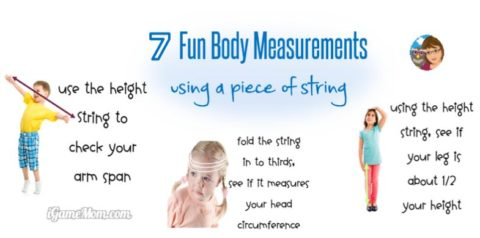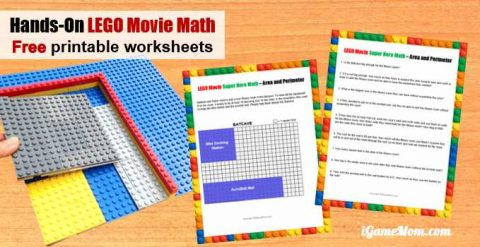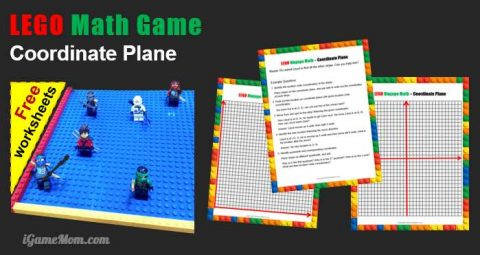# 3 Hands-On Activity to Learn about Pi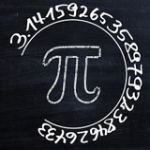Do you celebrate Pi Day? It is March 14 every year, since the number Pi is 3.14…… It is a great opportunity to do some fun math activities with kids. Here is a brief introduction of Pi and some quick Pi Day math activity ideas for kids of all ages. At the end of the post, you will find a free printable download to go with these activties, including the first 100 digits of the Pi, Pi and Pi Day information, a data recording sheet for calculating the Pi, and discussion questions after the activities.

## What is Pi?

Pi (π) is the ratio of a circle’s circumference to its diameter. It is a constant number, no matter the size of the circle.

The diameter of a circle is a straight line segment that passes through the center of the circle and ends on the circle. It is the longest line segment passes through a circle. The circumference of a circle is distance around the circle. It is the length of the circle if it were opened up and straightened out to a line segment.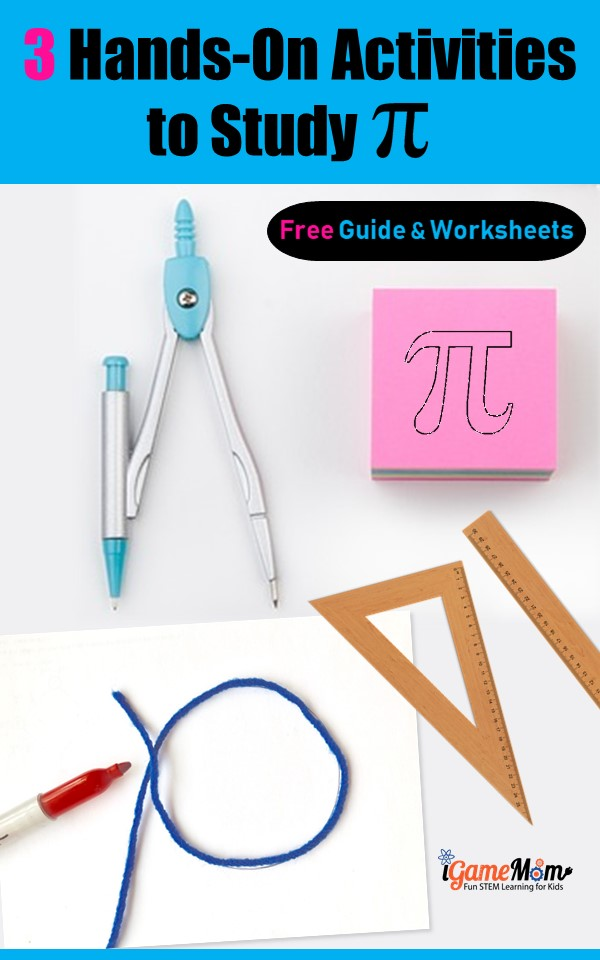## Why Do We Celebrate Pi Day?

The number Pi plays a significant role in mathematics. Since the first 3 digits of Pi are 3, 1, and 4, and the date March 14 coincident with the 3 digits (3.14), people around the world celebrate the day with pies and various math activities and events. It is a great way to engage kids and every one in math and science and have fun.

## First Pi Activity

Memorize Pi competition: see who can memorize the most digits of Pi. In the download package, you will have a sheet of the first 100 digits of Pi. Can you memorize all 100 digits?

Someone may argue that memorizing 100 digits of Pi is useless and is a waste of time. It might be true. However, many mental games are not directly useful in any way, but they train your brain power and enhance your number senses. Therefore, it is a fun math activity for Pi day.

Helpful tip: think them as 10 telephone numbers. It feels less danting when trying to remember 10 phone numbers vs memorizing 100 digits random numbers.

## Second Pi Activity

Calculate the Pi value: as we know Pi is the ratio of a circle’s circumference and diameter. No matter what is the size of the circle, the ratio is always the same. Can’t believe it? Try it out for your self.

2) In addition to a brief introduction of the Pi and a step-by-step instruction, in the package, you will find a measurement recording sheet. You will need the sheet to record your measurements and calculations.
3) Find another blank piece of paper and a compass to draw circles of different sizes. I suggest using a compass instead of any round shape object, because it is a lot easier to find the center of the circle, thus easier to measure the diameter of the circle, when you use a compass.
4) Use a thread to go around the full circle and then measure the thread to get the circumference of the circle.
Note: based on my experience, it is easier to cut out the circle, then run the thread through the edge of the circle.
5) Use a ruler to measure the diameter.
6) Record your measures of diameter and circumference for each circle on the recording sheet. There are spaces for 9 circles on the recording sheet. If you have more than 9 circles, please print another copy of the sheet.
7) Calculate Pi for each circle (Pi = circumference / diameter)
8) Compare the Pi’s from your calculation. Are the same?
9) Take a look at the reflection questions in the package. Can you answer those questions?
10) What is the value of the Pi?

## Third Pi Activity

Search your birthday in Pi: Pi is an irrational number, which means that its digits never end and that it doesn’t contain repeating sequences of any length. With this, it is very likely you can find a random number sequence in Pi.

To play Pi search game, go to this Pi Search website, put in your 2-digit birth month followed by your 2-digit birth day into the blank box after “Search For”, then click on “search pi”. You will see the digit position of your birthday number sequence.

Whose birthday in your group appears first in Pi?

Have fun!

For more hands-on math activities with Free printable worksheets and instructions, visit:
7 Fun Body Measurements with a Piece of String: fun non-standard measurement activity
LEGO Superhero Math for Area and Perimeter: learn area and perimeter with LEGO movie stories and visuals built with LEGO
LEGO Math for Coordinate Plane: practice ordered pair and coordinate plane with LEGO ninjas.
Proof Pythogorean Theorem with LEGO: visualize the Pythagorean Theorem with LEGO moves that even first graders can understand.